# Of Filters & Phase Relationships

Having dealt last month with the concepts of envelopes, oscillators and LFOs, Gordon Reid moves on to the subject of filters, and the havoc they wreak on the signals that pass through them.

So, you've read Parts 1, 2 and 3 of this series, and you've digested everything I've written so far about oscillators, envelope generators, VCAs, and LFOs, how they work, what they do, and how they slot into the typical subtractive synthesizer. About now, you imagine, it ought to be time to get the low-down on the crowning glory of subtractive synthesis — the filters. Well, yes... and no. If you're expecting something like "wind the resonance up to 11, and sweep the 24dB/octave VCF for some classic tearing analogue sounds," you're going to be disappointed.

If you really want to know why some filters sound good and others are so uninspiring, you've got to understand more about them. Unfortunately, filters are the most misunderstood parts of any synthesizer, and even the most basic belief surrounding them (that they just make parts of the signal quieter) is wrong. A filter — analogue or digital — does far more than just attenuate... it really screws with your signal.

To see exactly what happens when you pass a signal through a filter, it's necessary to know something about phase relationships. And to hack that, you need to take another look at the sine waves introduced in the first Part of this series.

## Just A Phase

Let's start by considering what happens when you combine two sine waves using a simple mixer. As you might imagine and as you can see in Figure 1, adding together two identical waves produces the same sound, but louder. But what happens if you start the lower wave halfway through the cycle of the upper one? Figure 2 shows that, if you add these waves together, they cancel each other out, and you hear nothing. Although, in isolation, these signals would sound identical, combining them results in silence.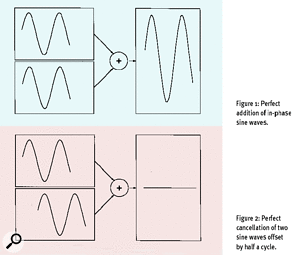Figure 1: [top] Perfect addition of in-phase sine waves. Figure 2: [bottom] Perfect cancellation of two sine waves offset by half a cycle.This is an important result, which demonstrates that while you can describe a single sine wave by specifying just its frequency and amplitude, if you combine two or more waves you must consider their relative offset. This offset is usually called the 'phase' of one wave with respect to the other, and is expressed in degrees, like the ones used to describe angles (if you want to know why, have a look at the 'Expressing Phase In Degrees' box below, but if you can't be bothered with another technical explanation at this point, read on).

Of course, you can mix sine waves with any offset (ie. any phase difference) and the amplitude of the result will lie somewhere between the 'double' loudness of Figure 1 and the silence of Figure 2 (if, instead of combining the signals in mono, you play the waves independently through stereo speakers, you'll get a quite different result. But that's a topic for another day, and we won't discuss it further here).

Instead, let's consider this offset as a timing difference. Let's say that our sine waves have a frequency of 100Hz or, to use older terminology, oscillate at a frequency of 100 cycles per second. We can say, therefore, that each cycle takes 0.01 seconds and, for these signals, an offset of half a cycle (a phase shift of 180º, if you've read that box yet, and if you haven't, don't worry) is equivalent to a time shift of 0.005 seconds or, as it is more commonly expressed, 5 milliseconds. Figure 3 should help to make this clearer.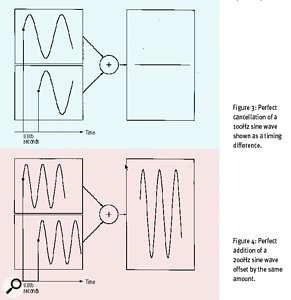Figure 3: [top] Perfect cancellation of a 100Hz sine wave shown as a 0.005 seconds timing difference. Figure 4: [bottom] Perfect addition of a 200Hz sine wave offset by the same amount.But now consider a different pair of sine waves at twice the frequency of the first. These have a frequency of 200Hz, and so five milliseconds is now sufficient time to complete a full cycle. In this case, as shown in Figure 4, our two sine waves add if one is delayed by 0.005 seconds.

This is because two signals that are offset by a complete cycle (360º out of phase) are once again perfectly in phase with each other!

Right, let's take a deep breath, and apply these ideas to a more complex waveform — say a sawtooth. As you may remember from the first Part of this series, a sawtooth wave has every harmonic present, so, if the fundamental (the first harmonic) lies at 100Hz, the second harmonic will be at 200Hz, the third at 300Hz... and so on. Adding two of these sawtooths (sawteeth?) with the fundamentals offset half a cycle means, of course, that the fundamentals are cancelled out. But the second harmonics, lying at 200Hz, will be added! The third harmonic, at three times the frequency of the fundamental, will be cancelled out, the fourth harmonic will be reinforced, the fifth cancelled... and so on. The result is a waveform with harmonics at 200Hz, 400Hz, 600Hz and so on... in fact, it's a sawtooth of the same amplitude but exactly twice the frequency of the originals.

This is a stunning result, quite at odds with much perceived wisdom. So this month's first Synth Secret is:

Combining complex 'out of phase' signals does not necessarily lead to complete cancellation. In fact, in the real world, it rarely, if ever, does so.

Yet even this result is one of the simplest cases of phase-shifting you can consider. Imagine how much more complex the result becomes when you apply these ideas to a complex waveform: some harmonics will be a bit quieter, some will be a bit louder, a few will be completely cancelled, and a few will be perfectly added. But this is, of course, what happens in the real world. Fourier analysis (back to Part one again, for anyone who can't remember) tells us that any two complex signals — such as those representing speech or music — can be described as an infinite number of sine waves that represent all the frequencies present in the signal. So, for any given offset between two otherwise identical signals, each frequency will be phase-shifted by a different amount. The result, when viewed on a spectral analyser, looks like a broad comb, with the distance between the 'teeth' of the comb (the cancellations) defined by the timing difference (see Figure 5, below).

In other words, when you mix two offset but otherwise identical signals, the phases of the individual frequencies define a filter. This, because of its characteristic shape, is called a Comb Filter, and you'll find examples of this on instruments as diverse as the Analogue Systems RS Integrator (a traditional modular analogue synth) and the DSP-based Waldorf Q.

## Phasing & Filtering

OK, so now you know something about phase, and you can see that this can be closely associated with audio filtering. But ask yourself this: If phase changes lead to filtering, can you assume that filtering leads to phase changes? The answer is, of course, yes.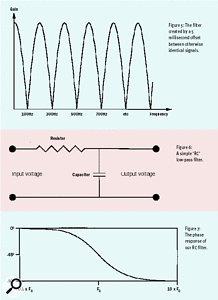Figure 5: [top] The filter created by a 5 millisecond offset between otherwise identical signals. Figure 6: [middle] A simple 'RC' low-pass filter circuit. Figure 7: [bottom] The phase response of our RC filter.Look at the electrical circuit in Figure 6. This has just two components — a resistor and a capacitor — but it depicts a perfectly usable filter called an RC low-pass filter. And, as any novice synthesist knows, a low-pass filter passes unhindered all the frequencies below a 'cutoff' frequency while attenuating all those above it. For this simple filter, the cutoff frequency is defined by the component values, while the nature of the circuit itself defines the rate at which the higher frequencies are attenuated.

Surprisingly (you may think) we're not going to worry about the rate of attenuation this month — we'll cover that next month. Instead, we're going to look at what this filter does to the phases of the signals fed into it...

Look at Figure 7 (bottom-right). This describes a property called the 'phase response' of our simple LPF, and it shows us that the phase of any given frequency presented at the filter's input will be shifted backwards to a greater or lesser extent. As you can see, low-frequency signal components are largely unaffected by the filter, a component at the cutoff frequency is shifted by exactly an eighth of a cycle ( or -45º), and high-frequency components are shifted by a full -90º.

Since these concepts are a bit esoteric (and because I want you to read to the end of this article without getting a headache) let's illustrate this by seeing what our RC filter does to something as fundamental to an analogue synth as a 100Hz square wave.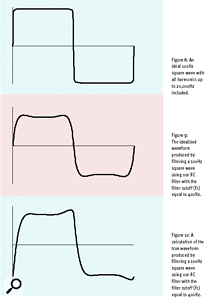Figure 8: [top] An ideal 100Hz square wave with all harmonics up to 20,000Hz included. Figure 9: [middle] The idealised waveform produced by filtering a 100Hz square wave using our RC filter with the filter cutoff (Fc) equal to 400Hz. Figure 10: [bottom] A calculation of the true waveform produced by filtering a 100Hz square wave using our RC filter with the filter cutoff (Fc) equal to 400Hz.If you recall Part 1 of Synth Secrets, you'll remember that you can represent any conventional waveform by a number of harmonics, called the fundamental and its overtones. In this case, our input signal (the square wave) has a fundamental of 100Hz. The second harmonic — at 200Hz — is absent, but the third harmonic lies at 300Hz and has an amplitude of a third of the fundamental. Similarly, the fourth harmonic is absent, but the fifth is present at 500Hz and has an amplitude of one-fifth... and so on. All the harmonics are in phase, and the waveform looks like that shown in Figure 8.

Now let's say that our simple RC filter has a cutoff frequency of 400Hz, and imagine what would happen to our square wave if the filter's phase response was zero at all frequencies. This is quite simple: the fundamental and first overtone of the square wave (the harmonics at 100Hz and 300Hz) would be unattenuated, but all the overtones at 500Hz and above would be attenuated according to the filter's response. The resulting waveform (and you'll have to trust me on this) is shown in Figure 9.

But now let's take into account the phase shifts imposed upon each of the harmonics in the signal. We now get a waveform that looks very different, and the true output from our filter (Figure 10) is visibly distorted when compared to the original.

This leads to a hugely important conclusion, and this month's most important Synth Secret:

Filters not only change a waveform by attenuation, but distort it by individually phase-shifting the harmonics within it.

Strangely, due to the relative simplicity of the filtered square wave, you probably won't be able to hear the difference between the waveforms in Figures 9 and 10 — you would need a more complex wave to hear the phase-shifting of the harmonics. But as you've already seen in this series, there are very few sounds in the real world that aren't more complex than a square wave, and so the effect on most sounds can be quite dramatic. And, of course, if it's a Moog filter you're passing the sound through... ah, but that's a discussion for another day.

## Expressing Phase In Degrees

How is it that we can express phase shifts in degrees? After all, degrees are used in maths to measure angles, or amounts of rotation, aren't they? Well, it all depends on how you look at things. Consider a simple sine wave — see (c). At some arbitrary time, the wave starts rising from zero, peaks exactly a quarter of the way through its cycle, crosses the zero line again halfway through, reaches its lowest point three-quarters of the way through the cycle, and then returns to zero before starting all over again.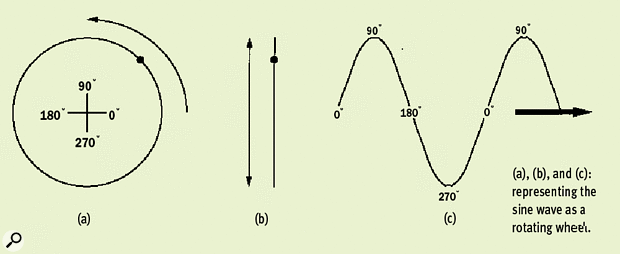The shape of the sine wave can, however, be described in a different way. Imagine a spot on the circumference of a wheel rotating at a constant speed, as shown in (a) — and imagine the shape it traces if you only consider the vertical element of its movement (this may seem like a rather arbitary thing to do, but trust me on this a bit longer). You'll get an up-and-down trace like the one shown in (b). Finally, imagine drawing that up-and-down motion on a piece of paper moving past it at a constant speed. Can you see that the result, shown in (c), would be a sine wave again? If not, blame my drawing skills (or lack thereof), because it is.

Working backwards, this approach allows us to employ the same units used to describe the rotation through which the wheel passes — degrees — to describe the progression through the sine wave cycle. The sine wave starts to be traced out when the spot on the circumference of the wheel is at the position that convention calls 0º. At the top of the cycle, the spot has rotated through 90º. It drops past zero again at 180º, has its lowest point at 270, and returns to the start after rotating a full 360º (which is the same thing as being at 0º again).

This is a very neat way of expressing this physical attribute of the waveform, and makes it easy to describe relative phase. For example, if two sine waves are offset by half a cycle with respect to each other, you can say that (because one is at 180º when the other is at 0º) these waves are '180º out of phase'.